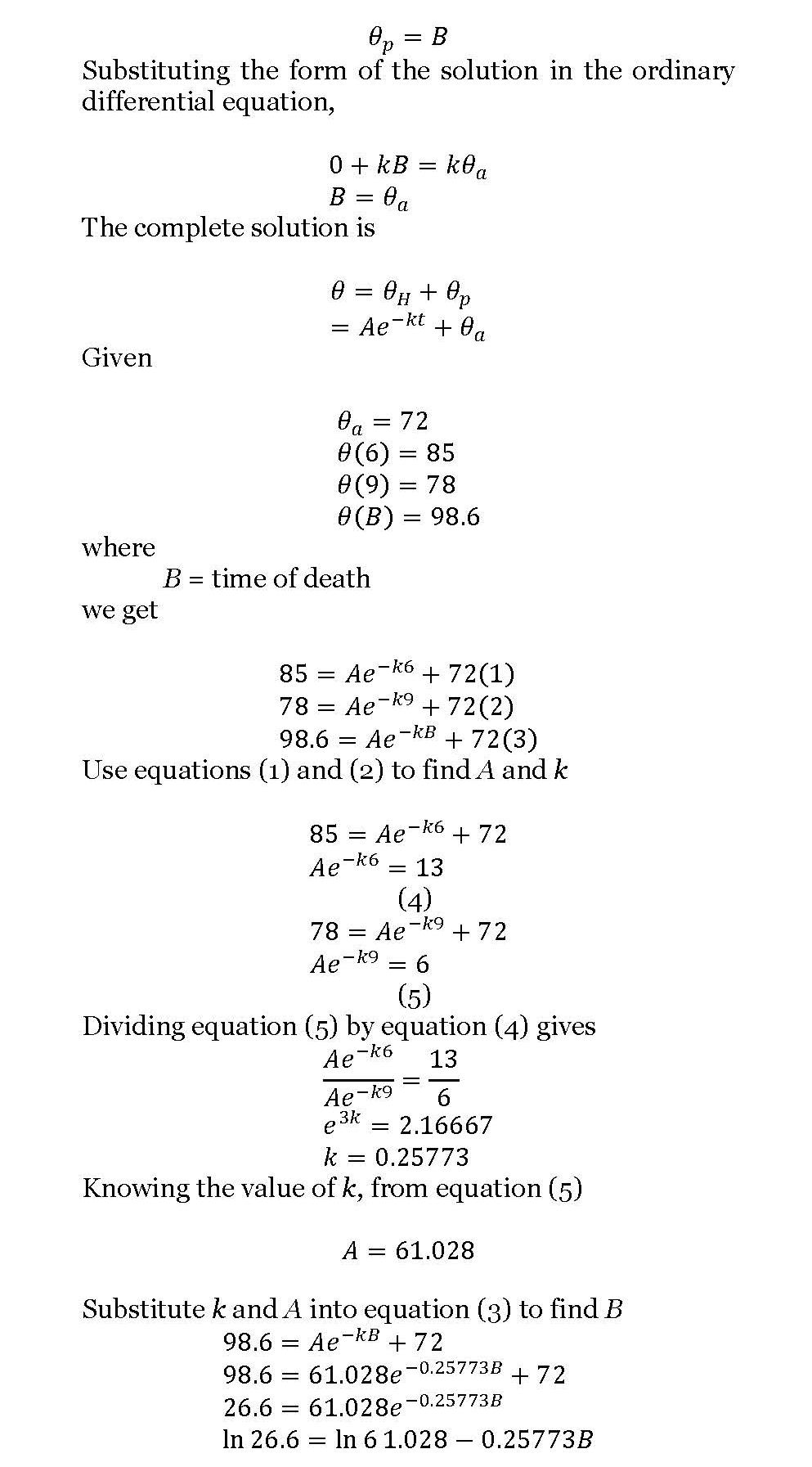## Time of death – a classic ODE problem

One of the classical applied problems in ordinary differential equations is that of finding the time of death of a homicide victim.

The estimation of time of death is generally based on the temperature of the body at two times – 1) when the victim is found and 2) then a few hours later. Assuming the ambient temperature stays the same and the body is treated as a lumped system, one can use a simple linear ODE to solve the problem.

It is somewhat an inverse problem as we are trying to find the value of the independent variable – the time of death. Here is a solved problem (a pdf version also available)._____________________________________________________

This post is brought to you by Holistic Numerical Methods: Numerical Methods for the STEM undergraduate at http://numericalmethods.eng.usf.edu